#### Venn Diagrams

A very important branch of mathematics with a wide variety of applications is Set Theory. It is a very basic and simple concept, but in Algebra, Logic, Combinatorics, Probability, etc., it has considerable use. Therefore, knowing this subject is of serious importance for the same purpose in solving different questions in competitive exams such as MBA entrance exams.

A basic representation of diagram sets is the Venn diagram, also known as the Euler-Venn diagram. For the sets under consideration, the regular representation makes use of a rectangle as the universal set and circles.

Distribution in competitive exams

CAT :

 Year No of Questions Good attempt Difficulty 2019 Slot 1 1 1 Moderate Slot 2 2 1 Difficult 2018 Slot 1 1 1 Easy Slot 2 1 0 – 1 Moderate
XAT :

 Year No of Questions Good attempt Difficulty 2020 2 2 Moderate 2019 2 1 Moderate

#### SNAP :

 Year No of Questions Good attempt Difficulty 2019 2 2 Easy-Moderate 2018 3 2 Moderate

### List of concepts in Venn Diagram

A rectangle representing the universal set starts with each Venn diagram. Then a circle represents each set of values in the problem. In the sections where the circles overlap, any values which belong to more than one set will be put.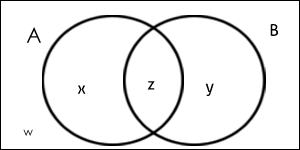### Solved Questions for Venn Diagram

Q1: In a college, 200 students are randomly selected. 140 like tea, 120 like coffee, and 80 like both tea and coffee.

• How many students like only tea?
• How many students like only coffee?
• How many students like neither tea nor coffee?
• How many students like only one of tea or coffee?
• How many students like at least one of the beverages?

The given information may be represented by the following Venn diagram, where T = tea and C = coffee.

Number of students who like only tea = 60

Number of students who like only coffee = 40

Number of students who like neither tea nor coffee = 20

Number of students who like only one of tea or coffee = 60 + 40 = 100

Number of students who like at least one of tea or coffee = n (only Tea) + n (only coffee) + n (both Tea & coffee) = 60 + 40 + 80 = 180

Q2. In a survey of 500 students of a college, it was found that 49% liked watching football, 53% liked watching hockey and 62% liked watching basketball. Also, 27% liked watching football and hockey both, 29% liked watching basketball and hockey both and 28% liked watching football and basketball both. 5% liked watching none of these games.

• How many students like watching all the three games?
• Find the ratio of the number of students who like watching only football to those who like watching only hockey.
• Find the number of students who like watching only one of the three given games.
• Find the number of students who like watching at least two of the given games.

n(F) = percentage of students who like watching football = 49%

n(H) = percentage of students who like watching hockey = 53%

n(B)= percentage of students who like watching basketball = 62%

n ( F ∩ H) = 27% ; n (B ∩ H) = 29% ; n(F ∩ B) = 28%

Since 5% like watching none of the given games so, n (F ∪ H ∪ B) = 95%.

Now applying the basic formula,

95% = 49% + 53% + 62% -27% – 29% – 28% + n (F ∩ H ∩ B)

Solving, you get n (F ∩ H ∩ B) = 15%.

Now, make the Venn diagram as per the information given.

Note: All values in the Venn diagram are in percentage.

The number of students who like watching all the three games = 15 % of 500 = 75.

The ratio of the number of students who like only football to those who like only hockey = (9% of 500)/(12% of 500) = 9/12 = 3:4.

The number of students who like watching only one of the three given games = (9% + 12% + 20%) of 500 = 205

The number of students who like watching at least two of the given games=(number of students who like watching only two of the games) +(number of students who like watching all the three games)= (12 + 13 + 14 + 15)% i.e. 54% of 500 = 270

Q3: Applicants for the doctoral programs of the Ambi Institute of Engineering (AIE) and Bambi Institute of Engineering (BIE) have to appear for a Common Entrance Test (CET). The test has three sections: Physics (P), Chemistry (C), and Maths (M). Among those appearing for CET, those at or above the 80th percentile in at least two sections, and at or above the 90th percentile overall, are selected for Advanced Entrance Test (AET) conducted by AIE. AET is used by AIE for final selection. For the 200 candidates who are at or above the 90th percentile overall based on CET, the following are known about their performance in CET:

1. No one is below the 80th percentile in all 3 sections.

2. 150 are at or above the 80th percentile in exactly two sections.

3. The number of candidates at or above the 80th percentile only in P is the same as the number of candidates at or above the 80th percentile only in C. The same is the number of candidates at or above the 80th percentile only in M.

4. Number of candidates below 80th percentile in P: Number of candidates below 80th percentile in C: Number of candidates below 80th percentile in M = 4:2:1.

BIE uses a different process for selection. If any candidate is appearing in the AET by AIE, BIE considers their AET score for final selection provided the candidate is at or above the 80th percentile in P. Any other candidate in P in CET, but who is not eligible for the AET, is required to appear in a separate test to be conducted by BIE for being considered for final selection. Altogether, there are 400 candidates this year who are at or above the 80th percentile in P.

If the number of candidates who are at or above the 90th percentile overall and also are at or above the 80th percentile in P in CET, is more than 100, how many candidates had to sit for the separate test for BIE?

It is given that 200 candidates scored above 90th percentile overall in CET. Let the following Venn diagram represent the number of persons who scored above 80 percentile in CET in each of the three sections:

From 1, h = 0.

From 2, d + e + f = 150

From 3, a = b = c

Since there are a total of 200 candidates, 3a + g = 200 – 150 = 50

From 4, (2a + f) : (2a + e) : (2a + d) = 4 : 2 : 1

Therefore, 6a + (d + e + f) is divisible by 4 + 2 + 1 = 7.

Since d + e + f = 150, 6a + 150 is divisible by 7, i.e., 6a + 3 is divisible by 7. Hence, a = 3, 10, 17, . . .

Further, since 3a + g = 5 0, a must be less than 17. Therefore, only two cases are possible for the value of a, i.e., 3 or 10.

We can calculate the values of the other variables for the two cases.

a = 3 or 10

d = 18 or 10

e = 42 or 40

f = 90 or 100

g = 41 or 20

Among the candidates who are at or above 90th percentile, the candidates who are at or above 80th percentile in at least two sections are selected for AET. Hence, the candidates represented by d, e, f, and g are selected for AET. BIE will consider the candidates who are appearing for AET and are at or above 80th percentile in P. Hence, BIE will consider the candidates represented by d, e, and g, which can be 104 or 80. BIE will conduct a separate test for the other students who are at or above 80th percentile in P. Given that there are a total of 400 candidates at or above 80th percentile in P, and since there are 104 or 80 candidates at or above 80th percentile in P and are at or above 90th percentile overall, there must be 296 or 320 candidates at or above 80th percentile in P who scored less than 90th percentile overall.

From the given condition, the number of candidates at or above 90th percentile overall and at or above 80th percentile in P in CET = 104. The number of candidates who have to sit for separate test = 296 + 3 = 299.

Q4. In an exam conducted for 38 students of class XII 15 passed in Biology and 25 passed in Chemistry If at least 1 student failed in both the subjects, find the maximum number of students who passed in the only Biology?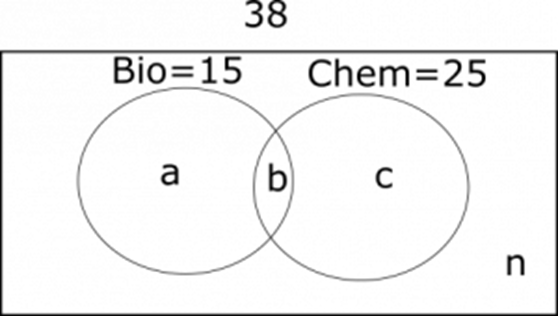From the Venn diagram , a + b = 15 – (1)

b + c = 25 – (2)

a + b + c + n = 38 – (3)

(3) – (2) – (1) = b – n = 2

as n ≥ 1, the minimum value of b is 3.

From (1) the maximum value of a is 12

Q5: Of 60 students in a class, anyone who has chosen to study maths elects to do physics as well. But no one does maths and chemistry, 16 do physics and chemistry. All the students do at least one of the three subjects and the number of people who do exactly one of the three is more than the number who do more than one of the three. What are the maximum and the minimum number of people who could have done Chemistry only?

Let us outline the diagram. Anyone who does Maths does Physics also.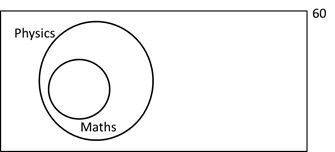Maths is a subset of Physics. Now, let us build on this.

No one does maths and chemistry, 16 do physics and chemistry.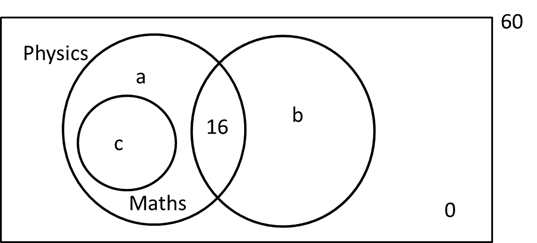Number outside is 0 as all the students do at least one of the three subjects

a + b + c +16 = 60, or a + b + c = 44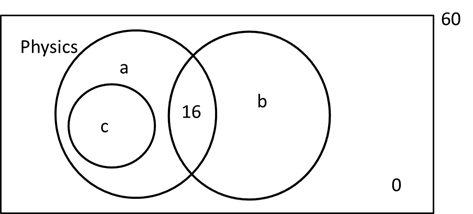The number of people who do exactly one of the three is more than the number who do more than one of the three. => a + b > c + 16
So, we have a + b + c = 44 and a + b > c + 16
We need to find the maximum and minimum possible values of b.
Let us start with the minimum. Let b = 0, a + c = 44. a > c + 16. We could have
a = 40, c = 4. So, b can be 0.
Now, thinking about the maximum value. b = 44, a = c = 0 also works.

So, minimum value = 0, maximum value = 44.

### How to prepare for CAT, XAT, GMAT, SNAP, and NMAT Venn Diagrams

Level 1 –

In the form of a Venn diagram, it is necessary to carefully list the conditions given in the query. Avoid taking several variables when solving such problems. Using the Venn diagram method and not with the aid of formulas, try answering the questions.

Level 2 –

To understand diagrams well, you must also practice. Arun Sharma’s ‘How to Prepare for LIDR for the CAT’ is known to be the best for CAT LIDR training. It is elaborate and easy to understand the examples given in it. To help you organize your training accordingly, it is also split into three stages of difficulty.

Level 3 –

Including all the above levels make sure you go through all the different Venn Diagram questions from the previous years of different exams, since so many questions are based on the concepts that have appeared in previous entrance exams. Spend lots of time per day to practice LIDR in general and measurement for the entrance test you intend to appear in particular. The more time you spend on the questions, the more familiar they’ll get.